Courses

# Doc: Representation of Rational Numbers on the Number Line Class 8 Notes | EduRev

## Class 8 : Doc: Representation of Rational Numbers on the Number Line Class 8 Notes | EduRev

The document Doc: Representation of Rational Numbers on the Number Line Class 8 Notes | EduRev is a part of the Class 8 Course Mathematics (Maths) Class 8.
All you need of Class 8 at this link: Class 8

Representation of rational numbers on number line:
A number that is expressed in the form a/b is called as rational number.
Here both "a" and "b" are integers and also b ≠ 0.
We all know, how to represent integers on the number line.
In the picture given below, the two integers 3 and 4 are represented on a number line.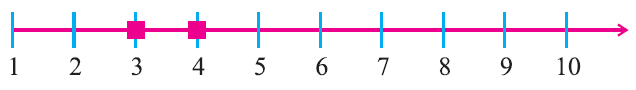In the picture given below, the integers -1, 0, 1, 2 and 3 are represented on a number line.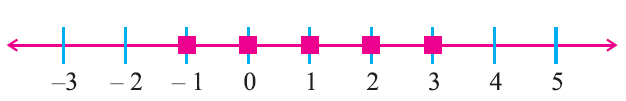On a number line, can you find any integer between 1 and 2 ?
No. But, between any two integers, we can represent rational rational numbers.
For example, between 0 and 1, we can represent rational numbers 1/10, 2/10, 3/10, .....which can be written as 0.1, 0.2, 0.3,.....Similarly, we know that the numbers 1/4, 1/2, 3/4 can be represented between 0 and 1. These are rational numbers which can be written as 0.25, 0.5, 0.75 respectively.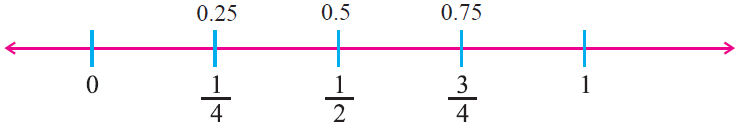Now, consider 2/5 and 4/5.
Can you find any rational number between 2/5 and 4/5 ?
Yes, There is a rational number 3/5.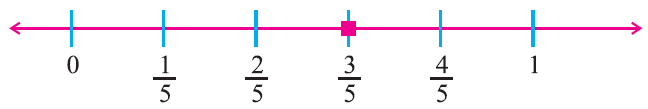In the same manner, we know that the numbers 1/5, 2/5, 3/5 and 4/5 are lying between 0 and 1.
Can you find more rational numbers between 2/5 and 3/5 ?
Yes. We write 2/5 as 20/50 and 3/5 as 30/50, then we can find many rational numbers between them.We can find nine rational numbers 21/50, 22/50, 23/50, 24/50, 25/50, 26/50, 27/50, 28/50 and 29/50.

If we want to find some more rational numbers between 22/50 and 23/50, we write 22/50 as 220/500 and 23/50 as 230/500.
Then we get nine rational numbers 221/500, 222/500, 223/500, 224/500, 225/500, 226/500, 227/500, 228/500 and 229/500.

Let us understand this better with the help of the number line in the figure given below.Observe the number line between 0 and 1 using a magnifying lens.
Similarly, we can observe many rational numbers in the intervals 1 to 2, 2 to 3 and so on.

Rational numbers between two rational numbers:
Let us consider the two rational numbers a/b and c/d. Here a, b, c and d are integers and also b ≠ 0, d ≠ 0.
We can find many rational numbers between a/b and c/d using the two methods given below.
1. Formula method
2. Same denominator method
Let us discuss these two methods in detail.
1. Formula method:
Let ‘a’ and ‘b’ be any two given rational numbers. We can find many rational numbers q1, q2, q3,...in between a and b as follows: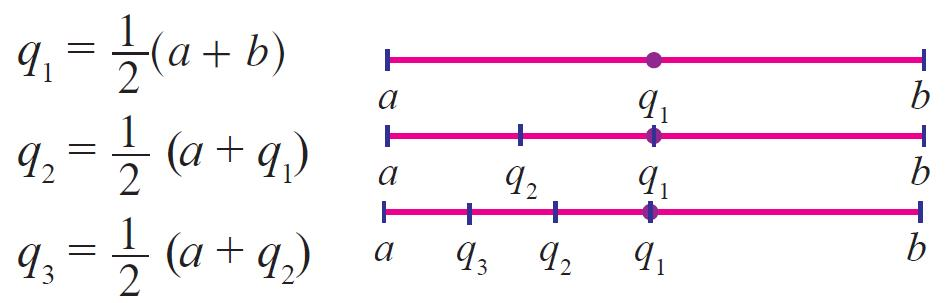The numbers q2, q3 lie to the left of q1. Similarly, q4, q5 are the rational numbers between ‘a’ and ‘b’ lie to the right of q1 as follows: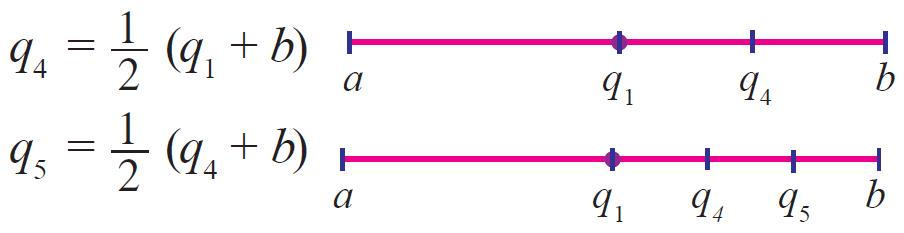Important note:
Average of two numbers always lies between them.

2. Same denominator method:
Let ‘a’ and ‘b’ be two rational numbers.
(i) Convert the denominator of both the fractions into the same denominator by taking LCM. Now, if there is a number between numerators there is a rational number between them.
(ii) If there is no number between their numerators, then multiply their numerators and denominators by 10 to get rational numbers between them.
To get more rational numbers, multiply by 100, 1000 and so on.
Important note:
By following different methods one can get different rational numbers between ‘a’ and ‘b’.

Example 1:
Find a rational number between 3/4 and 4/5.
Ans:
Formula method:
Let a = 3/4 and b = 4/5
Let q be a rational number between 3/4 and 4/5.
Then, we have
q = 1/2 x (a + b)
q = 1/2 x (3/4 + 4/5)
q = 1/2 x (15 + 16) / 20
q = 1/2 x 31/20
q = 31/40
Hence, the rational number between 3/4 and 4/5 is 31/40.

Same denominator method:
Let a = 3/4 and b = 4/5
L.C.M of the denominator (4, 5) is 20.
So, we can write "a" and "b" as given below
a = 3/4 x 5/5 = 15/20
and
b = 4/5 x 4/4 = 16/20
To find a rational number between 15/20 and 16/20 , we have to multiply the numerator and denominator by 10.
Then, we have
15/20 x 10/10 = 150/200
16/20 x 10/10 = 160/200
Therefore, the rational numbers between 150/200 and 160/200 are:
151/200, 152/200, 153/200, 154/200, 155/200, 156/200, 157/200, 158/200 and 159/200.

Example 2 :
Find two rational numbers between -3/5 and 1/2.
Ans:
Let a = -3/5 and b = 1/2
Let q1 and q2 be the rational number between -3/5 and 1/2.
First, let us get q1.
q1 = 1/2 x (a + b)
q1 = 1/2 x (-3/5 + 1/2)
q1 = 1/2 x (-6 + 5) / 10
q1 = 1/2 x (-1/10)
q= -1/20
Now, let's find q2
q2 = 1/2 x (a + q1)
q2 = 1/2 x (-3/5 - 1/20)
q2 = 1/2 x (-12 - 1) / 20
q2 = 1/2 x (-13/20)
q2 = -13/40
Hence, the two rational number are -1/20 and -13/40.

Note :
The two rational numbers can be inserted as:
-3/5 < -13/40 < -1/20 < 1/2.

Offer running on EduRev: Apply code STAYHOME200 to get INR 200 off on our premium plan EduRev Infinity!

## Mathematics (Maths) Class 8

212 videos|147 docs|48 tests

,

,

,

,

,

,

,

,

,

,

,

,

,

,

,

,

,

,

,

,

,

;